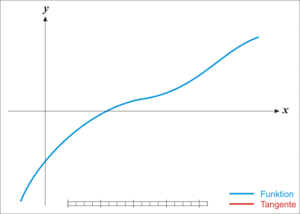•5星
389KB qq_44625365 2021-08-23 17:20:14
•5星
42.39MB qq_36749728 2021-06-26 16:16:18
•5星
1KB weixin_42696271 2021-09-11 04:51:07
•5星
600KB m0_52957036 2020-10-27 12:49:05
•5星
5.98MB weixin_44154817 2020-11-14 10:01:11
• X射线双能计算机层析成像投影分解的优化迭代方法 研究论文

1.87MB weixin_38660108 2021-03-29 02:32:55
• 15.53MB weixin_38680957 2021-01-26 08:55:32
• 10种优化迭代算法基础详解及其pytorch实现 机器学习 深度学习 python

优化器的功能：管理并更新模型中可学习参数的值，使得模型输出更接近真实标签。 这里的可学习参数一般就是指权值和偏置了，管理指优化器可以修改哪一部分参数。更新就是优化器的更新策略，每个不同的优化器会采取...

该篇笔记整理自余庭嵩的讲解。如果主要看优化器在pytorch中如何使用则可以直接看optim.SGD这一部分的讲解，该部分对优化器pytorch实现时的各变量解释详细，并且其他优化器的变量与其大同小异，在其他部分里就不再重新说明了。本文重点是探讨其中一种优化器的全部数学原理，其他优化器都是基于不同程度上的改进，最后一部分进行了罗列，如果需要详细了解原理可根据所给论文去阅读。

基本概念

优化器的功能：管理更新模型中可学习参数的值，使得模型输出更接近真实标签。
这里的可学习参数一般就是指权值和偏置了，管理指优化器可以修改哪一部分参数。更新就是优化器的更新策略，每个不同的优化器会采取不同的策略去更新参数的值，这里策略通常是梯度下降。
在展开讨论之前，先明确下面几个基本概念：

• 导数：函数在指定坐标轴上的变化率
• 方向导数：指定方向上的变化率
• 梯度：一个向量。方向为使得方向导数取得最大值的方向（各个方向上变化率最大的那个方向），模长就是这个最大的变化率。
• 梯度下降：一种策略。梯度是增长最快的方向，所以取梯度的负方向就是下降最快的那个方向。函数值就是loss值，所以用梯度下降来更新权值，使得函数值最快地降低。

PyTorch实现与机制

优化器的属性

在pytorch中优化器类名定义为Optimizer，其继承于object父类。优化器中包括如下几个主要基本属性：

• defaults：优化器的超参数。里面通常存储学习率，momentum的值以及decay的值等等
• state：用于存储参数的缓存。例如momentum会使用到前几次更新时所使用的梯度，那前几次的梯度就需要缓存在state中
• param_groups：管理的参数组。以list形式存储，list中的每一个元素是一个字典，字典的key（源代码里是’params’）对应的value才是真正的参数，这里的value其实又是一个list，里面包含了多个参数值。前面基本概念中提到了管理的概念，param_groups中存储的参数就是优化器可以修改的全部参数
• _step_count：记录更新次数，学习率调整中会使用到。例如100轮下降一次学习率，200轮再下降一次学习率，下降了几次就保存在这个属性中

优化器的方法

还包含如下几个主要的基本方法（函数）：

• step()：执行一步更新。当计算得loss，再用loss.backward()反向传播计算得到梯度之后，就需要使用step()进行权值参数的更新。更新的策略根据优化器的变化而变化。
• state_dict()：获取优化器当前状态信息字典。这个函数的返回值是一个字典，字典中只有两个key。一个是state，一个是param_groups。

优化器方法使用实例

首先我们手动创建一个可学习的参数，给其梯度赋值，然后定义好随机梯度下降优化器准备以其为例：

optimizer = optim.SGD([weight], lr=0.1)

之后我们依次观察优化器的每个方法都做了些什么。

optimizer.step()

通过下面代码构造使用实例

print("weight before step:{}".format(weight.data))
optimizer.step()        # 修改lr=1 0.1观察结果
print("weight after step:{}".format(weight.data))

输出结果为

weight before step:tensor([[0.6614, 0.2669],
[0.0617, 0.6213]])
weight after step:tensor([[ 0.5614,  0.1669],
[-0.0383,  0.5213]])

以第一个值为例，0.6614变成了0.5614，为什么？因为梯度设置的是1，学习率是0.1，所以一次更新1*0.1就等于0.1，由于更新策略定义了是随机梯度下降，所以要在梯度的反方向上更新，所以就在原权值的基础上减去0.1，由此得到最后结果。这里这么简单是因为我们直接赋值好了梯度，实际情况中梯度是要通过求解得出来的。具体的求解机制可粗浅理解入下，矩阵中每一个元素都影响着下一步的几个特定的输出，那矩阵中某个特定元素的梯度其实就是计算其影响的每个输出对其的偏导之和。
总结一下，step就是利用已经求好的梯度对权值进行一次更新。

通过下面代码构造使用实例

print("weight before step:{}".format(weight.data))
optimizer.step()
print("weight after step:{}".format(weight.data))
print("weight in optimizer:{}\nweight in weight:{}\n".format(id(optimizer.param_groups['params']), id(weight)))

输出结果为

weight before step:tensor([[0.6614, 0.2669],
[0.0617, 0.6213]])
weight after step:tensor([[ 0.5614,  0.1669],
[-0.0383,  0.5213]])
weight in optimizer:1587976042392
weight in weight:1587976042392

[1., 1.]])

tensor([[0., 0.],
[0., 0.]])

输出结果中值得注意的是，优化器中的权重地址和实际的权重地址是一样的，所以优化器中的权重和实际权重共享内存。

通过下面代码构造使用实例，给优化器以字典的形式添加一组参数

print("optimizer.param_groups is\n{}".format(optimizer.param_groups))

print("optimizer.param_groups is\n{}".format(optimizer.param_groups))

输出结果为

optimizer.param_groups is
[{'params': [tensor([[0.6614, 0.2669],
[0.0617, 0.6213]], requires_grad=True)], 'lr': 0.1, 'momentum': 0, 'dampening': 0, 'weight_decay': 0, 'nesterov': False}]
optimizer.param_groups is
[{'params': [tensor([[0.6614, 0.2669],
[0.0617, 0.6213]], requires_grad=True)], 'lr': 0.1, 'momentum': 0, 'dampening': 0, 'weight_decay': 0, 'nesterov': False},
{'params': [tensor([[-0.4519, -0.1661, -1.5228],
[ 0.3817, -1.0276, -0.5631],
[-0.8923, -0.0583, -0.1955]], requires_grad=True)], 'lr': 0.0001, 'momentum': 0, 'dampening': 0, 'weight_decay': 0, 'nesterov': False}]

就可以看见param_group中有两个字典了。

optimizer.state_dict()

通过代码构造下面使用实例：

optimizer = optim.SGD([weight], lr=0.1, momentum=0.9)
opt_state_dict = optimizer.state_dict()

print("state_dict before step:\n", opt_state_dict)

for i in range(10):
optimizer.step()

print("state_dict after step:\n", optimizer.state_dict())

torch.save(optimizer.state_dict(), os.path.join(DIR, "optimizer_state_dict.pkl"))

输出结果为

state_dict before step:
{'state': {}, 'param_groups': [{'lr': 0.1, 'momentum': 0.9, 'dampening': 0, 'weight_decay': 0, 'nesterov': False, 'params': }]}
state_dict after step:
{'state': {1636409304984: {'momentum_buffer': tensor([[6.5132, 6.5132],
[6.5132, 6.5132]])}}, 'param_groups': [{'lr': 0.1, 'momentum': 0.9, 'dampening': 0, 'weight_decay': 0, 'nesterov': False, 'params': }]}

可见，初次定义state_dict时，里面的state栏还没有信息。进行了十步更新之后，state_dict中就随时存储信息了。之后用torch.save把训练十步之后的优化器信息存在电脑硬盘上，后面就可以随时加载了。

通过代码构造下面使用实例，构建一个优化器，读取进来pkl，之后调用函数：

optimizer = optim.SGD([weight], lr=0.1, momentum=0.9)

输出结果为

{'state': {}, 'param_groups': [{'lr': 0.1, 'momentum': 0.9, 'dampening': 0, 'weight_decay': 0, 'nesterov': False, 'params': }]}
{'state': {2225753515928: {'momentum_buffer': tensor([[6.5132, 6.5132],
[6.5132, 6.5132]])}}, 'param_groups': [{'lr': 0.1, 'momentum': 0.9, 'dampening': 0, 'weight_decay': 0, 'nesterov': False, 'params': }]}

可见该函数加载进来了信息，可以接着这个信息进行训练了。

优化器数学原理

学习率

直接通过实例来理解学习率，例如目标函数如下：
y = 4 x 2 y=4x^2
把这里的x看成是权重，y看成是loss，下面通过代码来理解学习率的作用。
首先构造这个函数：

def func(x_t):

然后画出-10到10区间上的均匀分布的500个点的函数图像如下：接下来模拟一下优化的过程，假如不考虑学习率的概念，直接令其为1即可，迭代四次。

# 记录loss迭代次数，画曲线
iter_rec, loss_rec, x_rec = list(), list(), list()

lr = 1
max_iteration = 4

for i in range(max_iteration):

y = func(x)  # 得出loss值
y.backward()  # 计算x的梯度

x_rec.append(x.item())

# 更新参数

iter_rec.append(i)
loss_rec.append(y)

plt.subplot(121).plot(iter_rec, loss_rec, '-ro')
plt.xlabel("Iteration")
plt.ylabel("Loss value")

x_t = torch.linspace(-3, 3, 100)
y = func(x_t)
plt.subplot(122).plot(x_t.numpy(), y.numpy(), label="y = 4*x^2")
plt.grid()
y_rec = [func(torch.tensor(i)).item() for i in x_rec]
plt.subplot(122).plot(x_rec, y_rec, '-ro')
plt.legend()
plt.show()

观察loss值（也就是函数值的变化）：

Iter:0, X:     2.0, X.grad:    16.0, loss:      16.0
Iter:1, X:   -14.0, X.grad:  -112.0, loss:     784.0
Iter:2, X:    98.0, X.grad:   784.0, loss:   38416.0
Iter:3, X:  -686.0, X.grad: -5488.0, loss: 1882384.0

由此就引入了学习率的概念，一次更新先别更新那么多，只按着梯度的方向更新一小步，那怎么表示沿着梯度的方向更新一小步的概念？由此想到在梯度前面乘以数值对更新的程度进行缩小，这个数值就是学习率，如果令其为0.2，再更新四次，loss值和更新过程的曲线图如下所示：这就快要更新到那个最优值了，如果再增加迭代次数就变得收敛了，把迭代次数增加至20次，可得：那如果把学习率设置成0.125呢？这时候就会发现，只需要一步迭代，就直接迭代到了最优点，后面梯度为0，权值就不再更新了。那么由此就产生了疑问，不同的学习率对于优化的过程到底怎样的影响呢？为观察影响，设置不同的学习率来作出对比曲线图，看0.01到0.2上均匀分布的10个学习率对优化过程的影响曲线，代码如下：

iteration = 100
num_lr = 10
lr_min, lr_max = 0.01, 0.2  # .5 .3 .2

lr_list = np.linspace(lr_min, lr_max, num=num_lr).tolist()
loss_rec = [[] for l in range(len(lr_list))]
iter_rec = list()

for i, lr in enumerate(lr_list):
for iter in range(iteration):

y = func(x)
y.backward()

loss_rec[i].append(y.item())

for i, loss_r in enumerate(loss_rec):
plt.plot(range(len(loss_r)), loss_r, label="LR: {}".format(lr_list[i]))
plt.legend()
plt.xlabel('Iterations')
plt.ylabel('Loss value')
plt.show()

画出的对比曲线图为：可见，最小的学习率，收敛的时间也是最慢的，第二小的学习率，收敛时间第二慢。那么令收敛时间最快（也就是迭代次数最少）的学习率是哪一个呢？发现是0.136这条浅紫色的线。为什么？因为它距离0.125这个学习率最近，0.125是一步迭代直接就到最优值的那个学习率。
但是现实问题中，不会有人告诉你这个曲线的函数表达式让你求出来那个最快最合适的学习率。所以大部分情况只能设置0.01或者更小的学习率慢慢迭代，也可以达到收敛的效果。

动量

数学原理

结合当前梯度与上一次更新信息，用于当前更新。就是让更新的方向具有了惯性。在更加具体了解动量之前，先了解如下概念。
指数加权平均：一种求取平均值的方法
v t = β ∗ v t − 1 + ( 1 − β ) ∗ θ t \mathbf{v}_{t}=\boldsymbol{\beta} * \boldsymbol{v}_{t-1}+(\mathbf{1}-\boldsymbol{\beta}) * \boldsymbol{\theta}_{t}
这个方法的思想可以这么理解：要求取当前时刻的平均值，距离当前时刻越近的那些值，其参考性越大，其权重也就越大，而这些权重是随着时间间隔的扩大呈指数下降的。
看表达式内容， v t v_t 是当前时刻的值，它由两个部分组成，第一个部分是上一时刻的值，第二个部分是当前时刻的参数值，这两个部分加权求和就得到了当前时刻的平均值，这里权重之和为1。
举个具体实例说明一下。下图是一个时间序列，横轴是天数，纵轴是当天的温度：如果我需要求取第100天的时候的温度的平均值该怎么做？这时候就需要用到上面提出的指数加权平均公式了，代入公式如下：
v 100 = β ∗ v 99 + ( 1 − β ) ∗ θ 100 = ( 1 − β ) ∗ θ 100 + β ∗ ( β ∗ v 98 + ( 1 − β ) ∗ θ 99 ) = ( 1 − β ) ∗ θ 100 + ( 1 − β ) ∗ β ∗ θ 99 + ( β 2 ∗ v 98 ) = ( 1 − β ) ∗ θ 100 + ( 1 − β ) ∗ β ∗ θ 99 + ( 1 − β ) ∗ β 2 ∗ θ 98 + ( β 3 ∗ v 97 ) = ( 1 − β ) ∗ θ 100 + ( 1 − β ) ∗ β ∗ θ 99 + ( 1 − β ) ∗ β 2 ∗ θ 98 + ( β 3 ∗ v 97 ) = ∑ i N ( 1 − β ) ∗ β i ∗ θ N − i \begin{aligned} \mathbf{v}_{100}&=\beta * v_{99}+(1-\beta) * \theta_{100} \\ &=(1-\beta) * \theta_{100}+\beta *\left(\beta * v_{98}+(1-\beta) * \theta_{99}\right) \\ &=(1-\beta) * \theta_{100}+(1-\beta) * \beta * \theta_{99}+\left(\beta^{2} * v_{98}\right)\\ &=(\mathbf{1}-\boldsymbol{\beta})*\boldsymbol{\theta}_{\mathbf{1 0 0}}+(\mathbf{1}-\boldsymbol{\beta}) * \boldsymbol{\beta}*\boldsymbol{\theta}_{\mathbf{9 9}}+(\mathbf{1}-\boldsymbol{\beta})*\boldsymbol{\beta}^\mathbf{2}*\boldsymbol{\theta}_\mathbf{98}+\left(\boldsymbol{\beta}^\mathbf{3} * \boldsymbol{v}_\mathbf{97}\right)\\ &=(\mathbf{1}-\boldsymbol{\beta}) * \boldsymbol{\theta}_{\mathbf{1 0 0}}+(\mathbf{1}-\boldsymbol{\beta}) * \beta * \boldsymbol{\theta}_{\mathbf{9 9}}+(\mathbf{1}-\boldsymbol{\beta}) * \boldsymbol{\beta}^{\mathbf{2}} * \boldsymbol{\theta}_{\mathbf{9 8}}+\left(\boldsymbol{\beta}^\mathbf{3} * \boldsymbol{v}_\mathbf{97}\right)\\ &=\sum_{i}^{N}(\mathbf{1}-\boldsymbol{\beta}) * \boldsymbol{\beta}^{i} * \boldsymbol{\theta}_{\boldsymbol{N}-\boldsymbol{i}} \end{aligned}
这就可以发现，离当前时刻N越远的数值，权重是呈指数形式的，由于 β \beta 是一个小于1的数值，所以权重是呈指数下降的。那么下降程度到底是怎样的，可通过代码直观感受一下。

代码实例

首先构造一个上面公式里的函数，再给beta和N赋上具体数值：

def exp_w_func(beta, time_list):
# 返回多个时间点的权重值
return [(1 - beta) * np.power(beta, exp) for exp in time_list]

beta = 0.9
num_point = 100
time_list = np.arange(num_point).tolist()  # 100个时间点

接下来画出权重变化的图像：

weights = exp_w_func(beta, time_list)

plt.plot(time_list, weights, '-ro', label="Beta: {}\ny = B^t * (1-B)".format(beta))
plt.xlabel("time")
plt.ylabel("weight")
plt.legend()
plt.title("exponentially weighted average")
plt.show()

print(np.sum(weights))

得到图像可见，距离当前时刻越远，对于当前时刻平均值的影响就越小，越近，影响越大。这就是指数加权平均的思想。指数加权平均最核心的就是超参数 β \beta ，那么取不同值的时候权重是如何衰减的呢？
画出超参数 β \beta 分别为0.98,0.95,0.9,0.8时的对比图：

beta_list = [0.98, 0.95, 0.9, 0.8]
w_list = [exp_w_func(beta, time_list) for beta in beta_list]
for i, w in enumerate(w_list):
plt.plot(time_list, w, label="Beta: {}".format(beta_list[i]))
plt.xlabel("time")
plt.ylabel("weight")
plt.legend()
plt.show()

结果图为：通过这张图，对 β \beta 就可以理解为记忆周期的概念了， β \beta 越小，其记忆周期越短， β \beta 值越大，记忆周期越长。通常 β \beta 设置为0.9。因为更关注当前天数十天左右的数据。这个10是怎么来的呢？是根据以下公式得到的
l e n g t h = 1 1 − β length=\frac{1}{1-\beta}
那么为什么是这个公式呢？个人理解这就是当n很大的时候的等比数列求和公式，所有权值的之和会等于10，也就是综合考虑长度相当于10倍于当前天数的天数（10天）来给当前天数取值。

动量给SGD带来的改变

上面详细讨论的参数 β \beta 在pytorch的优化器中就是参数m也就是momentum系数。以随机梯度下降法为例，动量概念的引入到底可以给随机梯度下降法带来怎样的改变和优化呢？之前梯度下降更新权重的公式如下：
w i + 1 = w i − l r ∗ g ( w i ) \boldsymbol{w}_{\boldsymbol{i}+\mathbf{1}}=\boldsymbol{w}_{\boldsymbol{i}}-\boldsymbol{l} \boldsymbol{r} * \boldsymbol{g}\left(\boldsymbol{w}_{\boldsymbol{i}}\right)
其中，g是梯度。引入动量之后，pytorch中对权重的更新公式如下：
v i = m ∗ v i − 1 + g ( w i ) w i + 1 = w i − I r ∗ v i \begin{aligned} \boldsymbol{v}_{\boldsymbol{i}} =\boldsymbol{m} * \boldsymbol{v}_{\boldsymbol{i}-\mathbf{1}}+\boldsymbol{g}\left(\boldsymbol{w}_{\boldsymbol{i}}\right) \\ \boldsymbol{w}_{\boldsymbol{i}+\boldsymbol{1}} =\boldsymbol{w}_{\boldsymbol{i}}-\boldsymbol{I} \boldsymbol{r} * \boldsymbol{v}_{\boldsymbol{i}} \end{aligned}
其中v是更新量，m是momentum系数也就是参数 β \beta 。这里可以发现，不同之处在于更新权重时用v替换了梯度，而v，也就是更新量的大小和当前梯度以及之前的梯度都有关联。
还是举天数和气温的例子，第100天温度的平均值为：
v 100 = m ∗ v 99 + g ( w 100 ) = g ( w 100 ) + m ∗ ( m ∗ v 98 + g ( w 99 ) ) = g ( w 100 ) + m ∗ g ( w 99 ) + m 2 ∗ v 98 = g ( w 100 ) + m ∗ g ( w 99 ) + m 2 ∗ g ( w 98 ) + m 3 ∗ v 97 \begin{aligned} \boldsymbol{v}_{\mathbf{1 0 0}} &=\boldsymbol{m} * \boldsymbol{v}_{\mathbf{9 9}}+\boldsymbol{g}\left(\boldsymbol{w}_{\mathbf{1 0 0}}\right) \\ &=\boldsymbol{g}\left(\boldsymbol{w}_{\mathbf{1 0 0}}\right)+\boldsymbol{m} *\left(\boldsymbol{m} * \boldsymbol{v}_{\mathbf{9 8}}+\boldsymbol{g}\left(\boldsymbol{w}_{\mathbf{9 9}}\right)\right) \\ &=\boldsymbol{g}\left(\boldsymbol{w}_{\mathbf{1 0 0}}\right)+\boldsymbol{m} * \boldsymbol{g}\left(\boldsymbol{w}_{\mathbf{9 9}}\right)+\boldsymbol{m}^{2} * \boldsymbol{v}_{\mathbf{9 8}} \\ &=\boldsymbol{g}\left(\boldsymbol{w}_{\mathbf{1 0 0}}\right)+\boldsymbol{m} * \boldsymbol{g}\left(\boldsymbol{w}_{\mathbf{9 9}}\right)+\boldsymbol{m}^{2} * \boldsymbol{g}\left(\boldsymbol{w}_{\mathbf{9 8}}\right)+\boldsymbol{m}^{3} * \boldsymbol{v}_{\mathbf{9} \mathbf{7}} \end{aligned}

代码实例

对比一下有动量的SGD和没有动量的SGD的优化曲线，来产生直观的感受。代码如下：

def func(x):

iteration = 100
m = 0.

lr_list = [0.01, 0.03]

momentum_list = list()
loss_rec = [[] for l in range(len(lr_list))]
iter_rec = list()

for i, lr in enumerate(lr_list):

momentum = 0. if lr == 0.03 else m
momentum_list.append(momentum)

optimizer = optim.SGD([x], lr=lr, momentum=momentum)

for iter in range(iteration):

y = func(x)
y.backward()

optimizer.step()

loss_rec[i].append(y.item())

for i, loss_r in enumerate(loss_rec):
plt.plot(range(len(loss_r)), loss_r, label="LR: {} M:{}".format(lr_list[i], momentum_list[i]))
plt.legend()
plt.xlabel('Iterations')
plt.ylabel('Loss value')
plt.show()

先看都没有动量时候的loss值关于迭代次数的收敛曲线：再看看给0.01学习率情况添加动量后的对比曲线：从上图可以看见，添加了momentum的学习率为0.01时的SGD确实比没有momentum时候学习率为0.03时的SGD更快达到了最优值点。但是由于momentum设置得太大了，在到达最优值点的时候，算法还考虑了之前时刻的较大的梯度，所以虽然当前时刻梯度已经为0，但是更新量要考虑之前时刻的大梯度所以不为0，于是在最优点还进行了更新，误差就上去了。
那看看合适的momentum会是什么样的：PyTorch中的十种优化器

optim.SGD

随机梯度下降法，其具体原理可参考论文《On the Importance of Initialization and Momentum in Deep Learning》
主要参数：

• params：管理的参数组
• lr：初始学习率
• momentum：动量系数，也就是上面动量小节里面的 β \beta
• weight_decay：L2正则化系数的权重系数
• nesterov：是否采用NAG（参考文献《On the Importance of Initialization and Momentum in Deep Learning》），默认是false，一般不采用

optim.RMSprop

RMSprop结合Momentum，其具体原理可参考论文《Adam: A Method for Stochastic Optimization》

optim.ASGD

随机平均（Average）梯度下降，其具体原理可参考论文《Accelerating Stochastic Gradient Descent using Predictive Variance
Reduction》。

optim.Rprop

弹性反向传播，这个优化器的应用场景通常在用所有的样本一起计算梯度的情况下，也就是batchsize等于样本数的时候。这个情况叫做full batch，现在采用的都是mini batch。其具体原理可参考论文《Martin Riedmiller und Heinrich Braun》。

optim.LBFGS

BFGS的改进，L代表limited memory。是对BFGS在内存消耗上的一个改进。

展开全文nstarLDS 2020-03-14 19:43:01
• 1.46MB weixin_45776027 2020-06-01 10:24:16
• 672KB weixin_38632624 2021-05-13 01:45:44
• 683KB weixin_38536716 2021-05-20 05:39:52
• 769KB robotblog 2021-08-21 06:04:35
• 离散线性时不变系统的拟牛顿型优化迭代学习控制 研究论文

535KB weixin_38499553 2021-03-24 14:53:09
• 335KB weixin_38522552 2021-05-09 13:41:12
• 伪仿射投影算法优化迭代步长的研究 研究论文

666KB weixin_38591223 2021-02-09 20:21:15
• 169KB weixin_38648037 2021-02-11 10:31:27
• 459KB weixin_38631738 2021-04-27 05:48:40
• 325KB weixin_38735782 2021-05-16 07:09:44
• 1.23MB u013883025 2021-08-11 23:29:26
• 455KB dbnjzy 2021-09-15 16:18:47
• 基于梯度的权重更新优化迭代算法 梯度 迭代 优化

有时间参数 tt 参与的一般都为迭代式的算法 gt 表示当前时刻的梯度； γ,β：常数；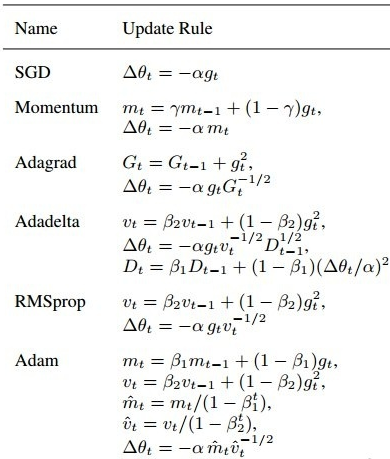• 有时间参数 t $t$ 参与的一般都为迭代式的算法
• gt$g_t$ 表示当前时刻的梯度；
• γ,β $\gamma, \beta$：常数；
展开全文lanchunhui 2017-03-20 18:44:37
• 如果学习机器学习算法，你会发现，其实机器学习的过程大概就是定义一个模型的目标函数J(θ)，然后通过优化算法从数据中求取J(θ)取得极值时对应模型参数θ的过程，而学习到的参数就对应于机器学习到的知识。...

目录(?)[+]

如果学习机器学习算法，你会发现，其实机器学习的过程大概就是定义一个模型的目标函数 J(θ) ，然后通过优化算法从数据中求取 J(θ) 取得极值时对应模型参数 θ 的过程，而学习到的参数就对应于机器学习到的知识。不管学习到的是好的还是无用的，我们知道这其中的动力引擎就是优化算法。在很多开源软件包中都有自己实现的一套优化算法包，比如stanford-nlp，希望通过本篇简要介绍之后，对于开源软件包里面的优化方法不至于太陌生。本文主要介绍三种方法，分别是梯度下降，共轭梯度法(Conjugate Gradient Method)和近似牛顿法(Quasi-Newton)。具体在stanford-nlp中都有对应的实现，由于前两种方法都涉及到梯度的概念，我们首先从介绍梯度开始。

什么是梯度，记忆中好像和高数里面的微积分有关。好，只要您也有这么一点印象就好办了，我们知道微积分的鼻祖是牛顿，人家是经典力学的奠基人，那么我们先来看看一道简单的物理问题：

一个小球在一个平面运动，沿着x轴的位移随时间的变化为： Sx=20t2 ，沿着y轴的位移随时间的变化为： Sy=10+2t2 ，现在求在 t0 时刻小球的速度 v

大家都是为高考奋战过的人，这样的小题应该是送分题吧。牛老师告诉我们，只要通过求各个方向的分速度，然后再合成就可以求解得出。好，现在我们知道各个方向的位移关于时间的变化规律，我们来求各个方向的速度。如何求速度呢，牛老师说位移的变化率就是速度，那么我们来求在 t0 时刻的变化率：

vx(t0)=limΔt>0(20(t0+Δt)2)(20t20)Δt=2t0
vy(t0)=limΔt>0(10+2(t0+Δt)2)(10+2t20)Δt=4t0
，那么此时的合成速度 v :
v=vx+vy=[2t0,4t0]
，此时的速度方向就是总位移变化最大的方向。搬到数学中，对于一个位移函数 S(x,y) ，它各个维度的变化率就是其对应的偏导数
S(x,y)x,S(x,y)y
，两者组合起来的向量就是该函数的梯度，所代表的含义上面已经说过，其方向代表函数变化最大的方向，模为变化率的大小。如果我们分别沿着 x,y 两个维度做微小的变化 Δx,Δy ,那么位移总体的变化将如下：
ΔS(x,y)S(x,y)xΔx+S(x,y)yΔy
现在我们知道如何求取函数的梯度，而且如何利用梯度求取函数微小变化量了。

梯度下降法

做机器学习(监督学习)的时候，一般情况是这样的，有 N 条训练数据 (X(i),y(i)) ，我们的模型会根据 X 预测出对应的 y ，也就是：

y(i)=f(X(i);θ)
其中 θ=[θ1,θ2,θ3,...,θn] 是模型的参数。通常我们希望预测值和真实值是一致的，所以会引出一个惩罚函数:
cost(y(i),y(i))
而目标函数则是：
J(θ)=i=0Ncost(y(i),y(i))=i=0Ncost(y(i),f(X(i);θ))
，我们目的是解决下面的优化问题:
argminθJ(θ)
，一般一组 θ N 条数据会对应一个 J(θ) ，也就是 N 维平面上的一个点，那么不同 θ 就可以得到一个 N 维的超平面(hyper plane)。特殊的假如 N=3 ，我们可能的超平面就如下图所示：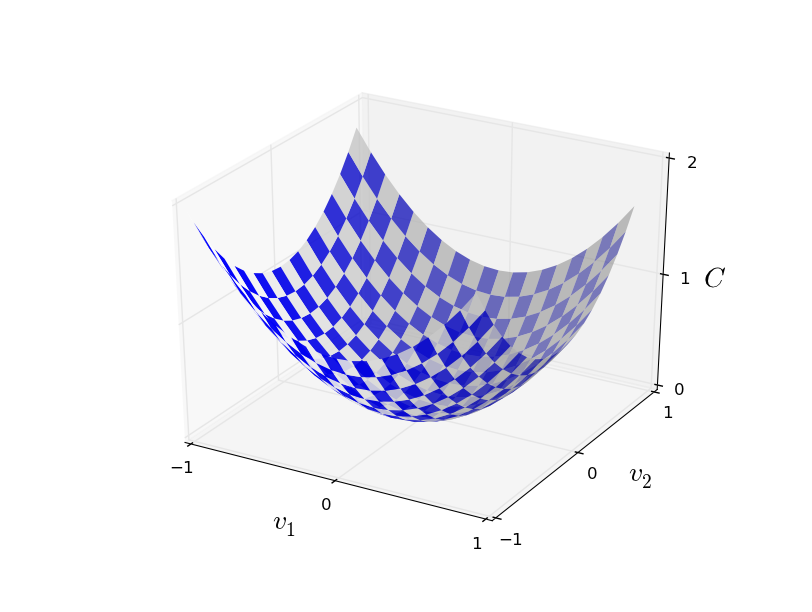如何找到最优的 θ 呢？一个想法是这样的：我们随机在超平面上取一个点，对应我们 θ 的初始值，然后每次改变一点 Δθ ，使 J(θ) 也改变 ΔJ(θ) ，只要能保证 ΔJ<0 就一直更新 θ 直到 J(θ) 不再减少为止。具体如下：

1. 随机初始化 θ
2. 对于每一个 θi 选择合适的 Δθi ，使得 J(θ+Δθ)J(θ)<0 ,如果找不到这样的 Δθ ，则结束算法
3. 对于每一个 θi 进行更新： θi=θi+Δθi ，回到第2步。

想法挺好的，那么如何找到所谓合适的 Δθ 呢？根据上一节中我们知道：

ΔJ(θ)iJ(θ)θiΔθi
,要如何保证 ΔJ(θ)<0 呢？我们知道，两个非0数相乘，要保证大于0，只要两个数一样即可，如果我们要保证 ΔJ(θ)>0 ，只要另每一个 θiJ(θ)θi 即可，此时
ΔJ(θ)iJ(θ)θiJ(θ)θi>0
，有人疑问了，我们目标可是要使 ΔJ(θ)<0 ，上面的做法刚好相反啊！反应快的人可能马上想到了，我们只需另每一个 θiJ(θ)θi 不就好了，而这样的求取向量 θ 各个维度相对于 J(θ) 的偏导数实际上就是求取 J(θ) 的梯度！回忆上一节梯度的含义，表示 J(θ) 变化最大的方向，想象一个球在上面的图中上方滚下来，而我们的做法是使他沿着最陡的方向滚。不错，我们找到了上述算法所说的合适的 Δθ 了！其实上述的算法就是我们本文的主角——梯度下降法(gradient descent)，完整算法如下：

1. 随机初始化 θ
2. 求取 θ 的梯度 θ ，也就是对于每个 θi 求取其偏导数 J(θ)θi ，并更新 θi=θiηJ(θ)θi(η>0)
3. 判断 θ 是否为0或者足够小，是就输出此时的 θ ，否则返回第2步

上述算法的第二步中多了一个未曾介绍的 η ，这是步伐大小，因为求取每一个维度的偏导，只是求取了该维度上的变化率，具体要变化多大就由 η 控制了， η 的选取更多考验的是你的工程能力，取太大是不可行的，这样导致算法无法收敛，取太小则会导致训练时间太长，有兴趣的可参考An overview of gradient descent optimization algorithms这篇文章中对 η 选取的一些算法。如何计算 J(θ)θi 呢？根据定义，可如下计算：

J(θ)θi=i=0Ncost(y(i),f(X(i);θ))θi

共轭梯度法

上一节中，我们介绍了一般的梯度下降法，这是很多开源软件包里面都会提供的一种算法。现在我们来看看另外一种软件包也经常见到算法——共轭梯度法(Conjugate Gradient Method)，Jonathan在94年的时候写过《An Introduction to the Conjugate Gradient Method Without the Agonizing Pain》详细而直观地介绍了CG，确实文如其名。这里我只是简要介绍CG到底是一个什么样的东西，具体还需阅读原文，强烈推荐啊！

最陡下降法(Steepest Descent)

上一节中，我们介绍了如何反复利用 θi=θiηJ(θ)θi 求得最优的 θ ，但是我们说选取 η 是一个艺术活，这里介绍一种 η 的选取方式。首先明确一点，我们希望每次改变 θ ，使得 J(θ) 越来越小。在梯度确定的情况下，其实 ΔJ(θ) 是关于 η 的一个函数：

ϕ(η)=J(θηθ)J(θ)=ΔJ(θ)
，既然我们想让 J(θ) 减小，那么干脆每一步都使得 |ΔJ(θ)| 最大好了，理论上我们可以通过求导求极值，令：
ϕ(η)=0
求得此时的 η ，这样每次改变 J(θ) 是最大的，而实际操作中，我们一般采用line search的技术来求取 η ，也就是固定此时的梯度 θ ，也就是固定方向，尝试不同的 η 值，使得
J(θηθ)
近似最小即可。这样固定方向的搜索和直线搜索没太大区别，也是名字的由来。表面看来，最陡梯度下降法应该是最优的啊，不但方向上是最陡的，而且走的步伐也是”最优”的，但是实际应用该方法的地方并不多，因为步伐的局部最优并不代表全局最优，导致其实际表现收敛速度比较慢(这却是优化算法的致命弱点！)。如果 J(θ) 是一个二次函数也就是 J(θ)=θTAθ+bTθ+c ，通过运行算法，我们可以得到一个如下的轨迹：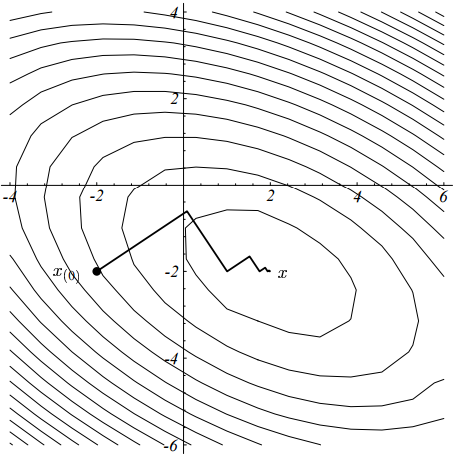我们可以发现，每一次走的步伐和上一次都是垂直的(事实上是可以证明的，在前面我推荐的文中有详细的证明:-))，这样必然有很多步伐是平行的，造成同一个方向要走好几次。研究最优化的人野心就来了，既然同一个方向要走好几次，能不能有什么办法，使得同一个方向只走一次就可以了呢？

共轭梯度法

Cornelius，Magnus和Eduard经过研究之后，便设计了这样的方法——共轭梯度法。具体详细的原理还是强烈推荐《An Introduction to the Conjugate Gradient Method Without the Agonizing Pain》一文，这里我只是利用文章中的思路进行简要介绍。

何谓共轭(Conjugate)

查看维基的介绍，共轭梯度法(CG)最早的提出是为了解决大规模线性方程求解，比如下面形式:

Ax=b
，其中 A 是方形对称半正定的。如果 A 越大并且越稀疏，导致其他线性方程求解不可行的时候，CG方法就更奏效。
我们已经了解梯度为何物，现在就差修饰词共轭(Conjugate)了，那么何为共轭(conjugate)呢？对于两个非零向量 d(i),d(j) ,如果满足
我们就称 d(i),d(j) 是关于 A 共轭的，也就是说其实共轭不共轭是相对于 A 而言。我们知道，如果两个向量正交，他们的内积为0，所以如果两个向量关于 A 是共轭的，我们也可以称这两个向量关于 A 是正交的，也就是 Aorthogonal

共轭梯度法求解线性方程组

那么求解上面线性方程组的时候，假如我们已经找到 n 个两两共轭的方向 {d(i)} ，如果将这些方向作为基，也就可以将 Ax=b 的解表示为 d(i) 的线性组合：

x=inαid(i)
如果左右分别乘上 A :
，也就是 αk=dT(k)bdT(k)Ad(k) 。现在问题就只在于如何找到这些神奇的共轭方向了，神奇之处在于这些共轭方向可以利用迭代方式求取，可以一次只求一个这样的方向向量 d(k) ，这也是该算法的核心之处。如果令 rk=bAxk ,那么
d(k+1)=rk+βkd(k)
其中 βk=rTk+1rk+1rTkrk
上一小节中利用Steepest Method的优化问题如果利用CG就变成了如下：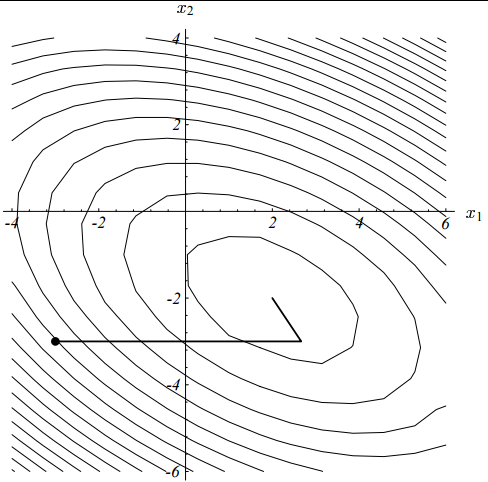二维的情况下，可以保证只走两步就达到收敛(严格的证明请参靠推荐的论文)！

非线性共轭梯度法

机器学习算法中，我们碰到的大部分问题都是非线性的，上面我们只是讲解了线性共轭梯度法，那么它可以解决非线性优化问题吗？很遗憾，不行，但是经过修改，可以利用共轭梯度法求取局部最优解，下面展示非线性共轭梯度法的大致轮廓,对于一个非线性目标函数 J(θ)

• 随机初始化 θ0 ，并令 r0=d(0)=J(θ0)
• 对于k = 0,1,2….
• 利用line search找出使得 J(θk+αid(k)) 足够小的 αk
• θk+1=θk+αkd(k)
• rk+1=J(θk+1)
• d(k+1)=rk+1+βk+1d(k)

这里又出现了 βi ,对于 β 的研究，著名的方法有:Hestenes-Stiefel方法、Fletcher-Reeves方法、Polak-Ribiére-Polyak 方法和Dai-Yuan方法，分别对应于：

βHSk=(rk+1rk)Trk+1dT(k)(rk+1rk)
βFRk=||rk+1||2||rk||2
βPRPk=(rk+1rk)Trk+1||rk||2
βDYk=||rk+1||2dT(k)(rk+1rk)

需要注意的是，非线性共轭梯度法并不能像解决线性系统那样，保证 n 步内收敛，一般我们迭代很多次直到 ||rk||<ϵ||r0|| 。
像CG这样高效的方法，一般都有现成的工具库可以使用，只要我们提供目标函数的一次导函数和初始值，CG就能帮我们找到我们想要的了！再次推荐《 An Introduction to the Conjugate Gradient Method Without the Agonizing Pain》一文。

近似牛顿法(Quasi-Newton)